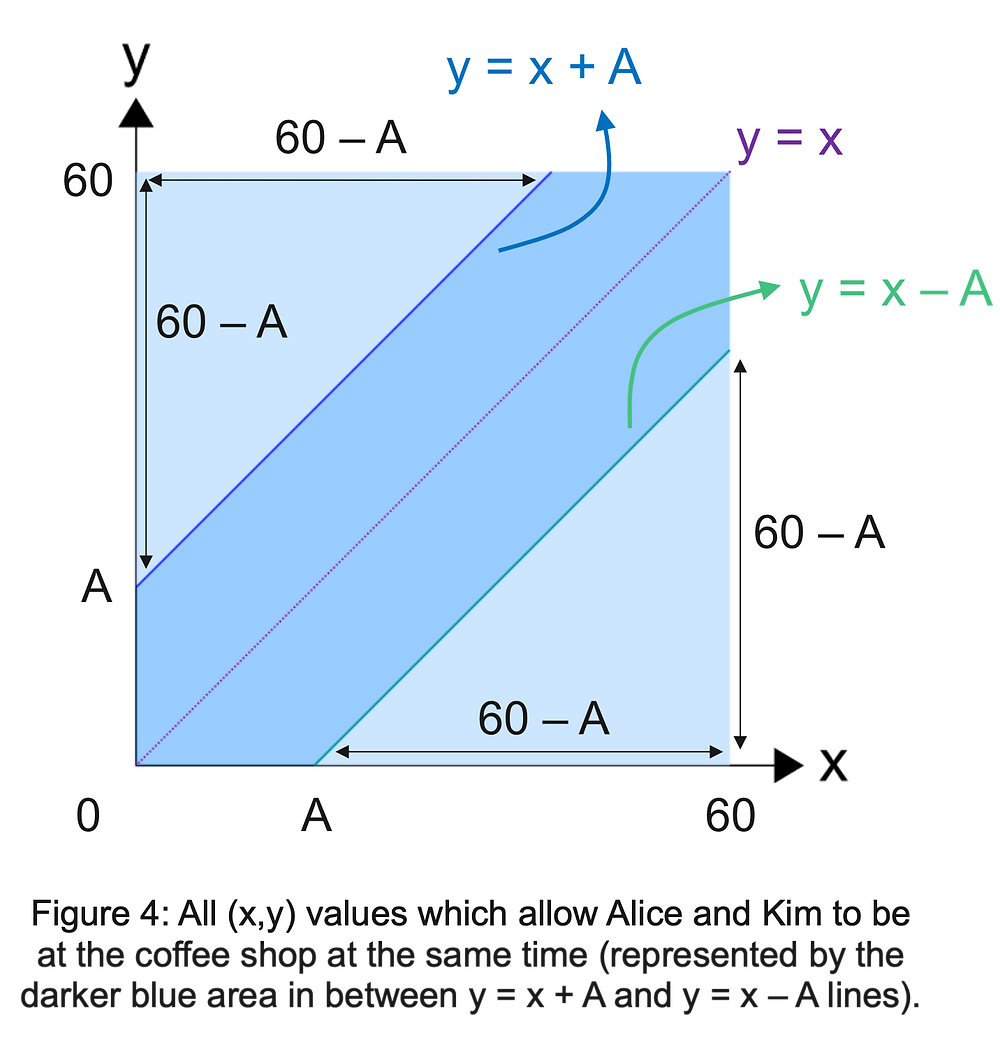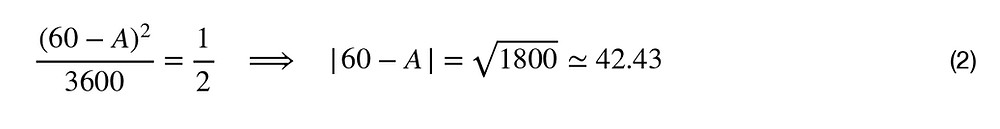Search

# Probability of seeing your friend

Updated: Feb 13

Two best friends, Alice and Kim, will go to the same coffee shop anytime between 2pm and 3pm on the same day, and each one will stay there A minutes and then leave. None of them will be there before 2pm and none of them will stay there after 3pm. If the probability for them to meet at the coffee shop between 2pm and 3pm is 50%, what is A?## SOLUTION:

Let’s call the time of arrival at the coffee shop for Kim as x and for Alice as y, respectively. Then, both x and y can take values between 0 and 60 minutes.Then, both x and y can take values between 0 and 60 minutes. Hence, we have chosen our starting time as t=0, corresponding to 2pm. Since, x and y are independent of each other, we can simply set them as two separate coordinate axes as shown in Figure 1. Then, the shaded area in Fig.(1) actually gives us all possible arrival time combinations, i.e (x,y) for these two best friends, so it is simply our sample space.

There are now two cases that we need to consider. Kim can arrive at the coffee shop before Alice (Case 1: x<y), or Alice can arrive at the coffee shop before Kim (Case 2: x>y).

CASE 1: Kim arrives first x < ySince, we know that Kim will stay at the coffee shop for A minutes, she will leave the coffee shop when the time is x + A min. So, if Alice arrives later than x + A, i.e y > x + A, they can not be there at the same time. So, the area on the sample space which satisfies the condition x < y < x + A represents the options for Alice and Kim being at the coffee shop at the same time, assuming Kim arrives earlier than Alice. This area is nothing but the region in between y = x and y = x + A lines as shown in Fig. (2).

CASE 2: Alice arrives first y < xSimilar to Case 1 now we will have the condition (replace x with y and y with x)

y < x < y + A which defines an area on the sample space, representing the possible (x,y) arrival time combinations for which Alice and Kim would be at the coffee shop at the same time, assuming Alice arrives earlier than Kim. The area of interest, then, is now between the lines

y = x and y = x – A as shown in Fig. (3).

Thus, the total probability for Kim and Alice to be at the coffee shop at the same time should be the ratio of the areas (darker blue) in Fig. (2) and Fig. (3) combined to the total area of the sample space given in Fig. (1). It is all summarized in Fig. (4).As is obvious from Fig. (4), the probability will definitely be a function of A. Using the geometry in Fig. (4), we find the ratio of the shaded area (darker blue) to the total area of the sample space asWe know that the probability for them to be at the coffee shop at the same time is 50%. This yieldsSince, we know that A < 60, we can find thatSo, if each one waits 17.57 minutes after arriving at the coffee shop, there will be a 50% probability that they will be at the coffee shop at the same time between 2pm and 3pm on that day.

Below shows the plot of P(A) as a function of A, as given in Eq. (1).Copyright © 2021 Ashlar STEM Academy

51 views0 comments

### Recent Posts

See All

#### MINIMUM WHOLE NUMBER VALUE

ARITHMETIC MEAN vs GEOMETRIC MEAN Copyright © 2021 Ashlar STEM Academy

#### Probability to form a Triangle by breaking a Rod